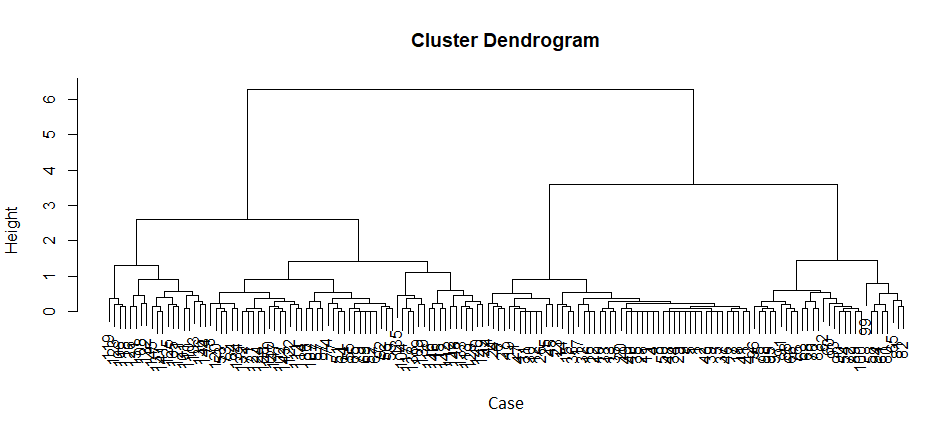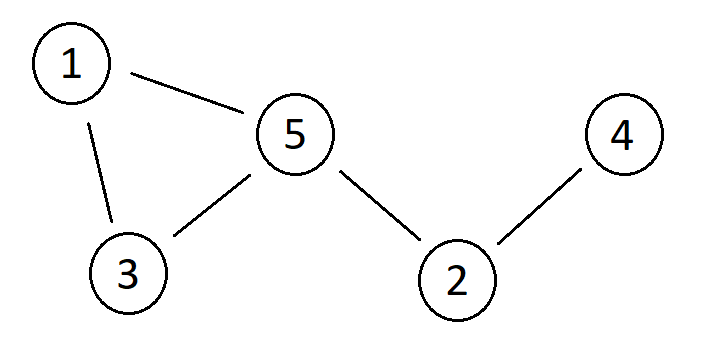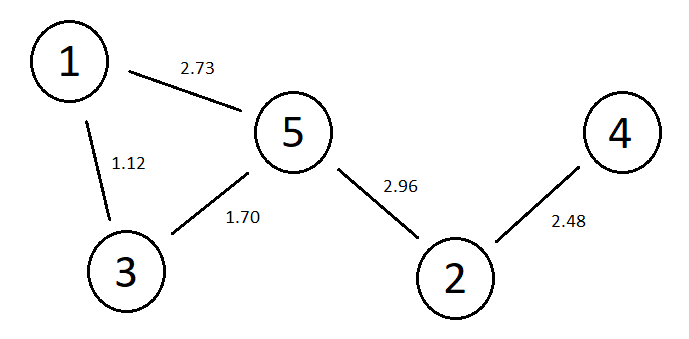•We examine sequential, hierarchical and optimization-based clustering approaches, including a section on graph-based clustering methods.
In this article, we will introduce common approaches to clustering algorithms: sequential, hierarchical and optimization-based. The sequential and hierarchical approaches do not fit cleanly into the definition of statistical models we gave in the first module of this course. Proximity functions play a central role in all these clustering approaches, and we will begin by defining what these functions are. We will then look at the the two approaches individually, explain their basic concepts and give basic algorithms for each. We will also look briefly at graph-based clustering methods that can be used with these techniques.
The optimization-based approach matches our definition of statistical models, and it is no surprise that the dominant tools in such methods are statistical and probabilitistic in nature. We will look at some important examples of optimization based clustering in the following steps.

## Proximity Measures

Proximity measures come in two forms: Dissimilarity and similarity measures. They give a measure of difference between two vectors, between one vector and one set of vectors, and between two sets of vectors. We begin with the case of proximity between two vectors, and provide as concrete examples the most common family of dissimilarity measures, $$l_p$$measures, and two common, and related, similarity measures, the inner product and cosine similarity measures.

### $$l_p$$ Dissimilarity Measures

Let $$X$$ and $$Y$$ be two vectors.
$d_p(X,Y)=\big(\sum_{i=1}^l w_i | X_i – Y_i|^p \big)^{1/p}$
Where $$w_i = 1$$ for $$1 \leq i \leq l$$ these are called unweighted $$l_p$$ measures. The unweighted $$l_2$$ measure is Euclidean distance. The unweighted $$l_1$$ measure is the Manhattan distance. The weights are used to make certain directions more important than others when calculating dissimilarity.

### Inner Product Similarity Measure

$s_{inner}(X_i,X_j)=X_i^TX_j$

### Cosine Similarity Measure

$s_{inner}(X_i,X_j)=\frac{X_i^TX_j}{\|X_i\| \|X_j\|}$

## Extending proximity measures to sets of vectors

Proximity measures between two vectors are simple to extend to cases between vectors and sets of vectors, and between two sets of vectors, using min and max (and mean, though this is less common) functions. Let $$d$$ be some proximity measure defined on vectors, and $$\Gamma$$ and $$\Delta$$ be two sets of vectors:
$d_{min}(X,\Gamma)=min_{Y \in \Gamma}(d(X,Y))$ $d_{max}(X,\Gamma)=max_{Y \in \Gamma}(d(X,Y))$ $d_{min}(\Gamma,\Delta)=min_{X \in \Gamma,Y \in \Delta}(d(X,Y))$ $d_{max}(\Gamma,\Delta)=max_{X \in \Gamma,Y \in \Delta}(d(X,Y))$
Alternatively, some representor for the cluster, $$R(\Gamma)$$ may be used. A common representor is the mean value of the vectors. In which case, we would have:
$d_{rep}(X,\Gamma)=d(X,R(\Gamma))$ $d_{rep}(\Gamma,\Delta)=d(R(\Gamma),R(\Delta)$

#### Euclidean Distance and High Dimensionality

It is common when working with a large number of dimensions (i.e. many columns in the data) to avoid $$l_p$$ dissimilarity measures such as Euclidean distance, and prefer alternatives such as cosine similarity.
This is because such dissimilarity measures fail to be discriminative as the number of dimensions increase. To explain things in a very hand-waving fashion, as the number of dimensions increase, points (from finite samples from some distribution) end up getting farther away from each other. The result is that in high dimensions, all points are a long way from all other points, and the difference in distances from a point to other points becomes a less useful way of distinguishing similar from dissimilar pairs. (In the general $$l_p$$ case, some researchers who have paid a lot of attention to this phenomenon claim that you can avoid this if you reduce $$p$$ fast enough as you increase dimensions, so you end up working with very small, fractional $$p$$ values. Most practitioners just use cosine similarity.)
If this seems odd to you, don’t worry – it seems odd to everyone. It turns out that our intuitions about distances that we have from visualizing the behaviour of sampling from distributions in one, two or three dimensions simply do not serve us very well in higher dimensions.

## Sequential Clustering

Sequential clustering algorithms are the simplest type of clustering algorithms, and are typically very fast to run. The basic sequential clustering algorithm is:
For some specified threshold, $$\theta$$, some dissimilarity measure $$d$$ (alternatively, some similarity measure, $$s$$) and, optionally, some limit on number of clusters, $$q$$:
Setup:
$m=1$ $C_m={X_1}$
Do:
• For $$i=2,…,N$$:
• Find $$C_k$$, such that $$k = arg min_{1 \leq j \leq m} d(X_i,C_j)$$ ($$k = arg max_{1 \leq j \leq m} s(X_i,C_j)$$)
• If $$d(X_i,C_k) \geq \theta$$ ($$s(X_i,C_k) \leq \theta$$) and $$m<q$$ then set $$m=m+1$$, $$C_m={X_i}$$
• Otherwise set $$C_k=C_k \cup X_i$$
Characteristics of this algorithm are:
• A single clustering is returned
• The number of clusters present is discovered by the algorithm
• The result is dependent on the order the data vectors are presented

## Hierarchical Clustering

Hierarchical clustering algorithms iteratively combine or divide clusters with use of the chosen proximity measure.

### Agglomerative Hierarchical Clustering

1. Initialize clusters such that $$C={C_i : 1\leq i \leq N}$$, where $$C_i = {X_i}$$, for $$1 \leq i \leq N$$.
2. m=N
3. While $$m>1$$:
1. Find $$C_j,C_k$$ such that $$arg min_{j,k} d(C_j,C_k)$$ ($$arg max_{j,k} s(C_j,C_k)$$), $$1 \leq j \leq m$$, $$1 \leq k \leq m$$, $$j \neq k$$.
2. Set $$C_j=C_j \cup C_k$$, $m=m-1$ and remove $$C_j$$ from $$C$$.

### Divisive Heirarchical Clustering

Divisive clustering works analagously to agglomerative clustering, but Begins with all data vectors in a single cluster which is then iteratively divided until all vectors belong to their own cluster.
Let $$S(C_i)=\{\langle \Gamma, \Delta \rangle : \Gamma \cup \Delta = C_i \land \Gamma \cap \Delta = \emptyset \land \Gamma, \Delta \neq \emptyset\}$$. In words, $$S(C_i)$$ gives all binary splits of $$C_i$$, such that neither resulting subset is empty.
1. Initialize clusters such that $$C={C_1}$$, where $$C_1 = {X_i : 0 \leq i \leq N}$$.
2. m=1
3. While $$m<N$$:
1. Find $$C_i, \langle \Gamma,\Delta \rangle$$ such that they are the solution to $$argmax_{C_i,\Gamma,\Delta} d(\Gamma,\Delta)$$ where $$\Gamma, \Delta \in S(C_i)$$ (alt. $$argmin_{C_i,\Gamma,\Delta} s(\Gamma,\Delta)$$ where $$\Gamma, \Delta \in S(C_i)$$)
2. Set $$C= (C \setminus C_i) \cup \{\Gamma,\Delta\}$$
Characteristics of both types of hierarchical algorithms are: – A heirarchy of clusterings is returned – The final clustering is not chosen by the algorithm

### Dendrograms

If we record the proximity values whenever clusters are divided or split in the above hierarchical algorithms, we can form a tree structure known as a dendrogram. A dendrogram gives an overview of the different sets of clusters existing at different levels of proximity. An example is given below.The above dendrogram has a property called monotonicity, meaning that each cluster is formed at higher dissimilarity level than any of its components. Not all clustering algorithms will produce monotonic dendrograms.

### Selecting a clustering from the hierarchy

Since hierarchical clustering algorithms do not provide a single clustering but rather a hierarchy of possible clusterings, a choice is required about which member of the hierarchy should be selected.
Visual or analytic analysis of dendrograms can be used to make this decision. In this case, an important concept is the lifetime of the cluster, where this is the difference between the dissimilarity levels at which a cluster is merged with another and the level at which it was formed. We would seek to use the clusters present at a level of dissimilarity such that all clusters existing at that level have long lifetimes.
Another option is to measure the self-proximity, $$h$$, of clusters, making use of set vs set proximity measures. For example, we might choose to define self-similarity as:
$h(C)=max_{X,Y \in C} \big(d_{l_2} (X,Y)\big)$
Where we remember that $$d_{l_2}$$ is Euclidean distance. We would then have to specify some threshold value $$\theta$$, for self-similarity such that we take the clustering at level $$L$$ in the hierarchy if at level $$L+1$$ there exists some cluster, $$C_i$$ such that $$h(C_i)>\theta$$. In this and the following paragraph we treat the first layer in the hierarchy as the clustering where all vectors are given their own cluster, and the last when all vectors are assigned to the same cluster.
A popular choice is to try and read the self-similarity threshold from the data. For example we might choose the largest layer such that the following condition is fulfilled:
$d(C_i,C_j) \geq max\big(h(C_i),h(C_j)\big)$
In words, the last layer where the dissimilarity of each pair of clusters is greater or equal to the self similarity of each of the clusters in the pair.

## Graph Clustering

To look at basic graph clustering, we need to introduce a number of concepts. Firstly, a graph, $$G=\langle N,E\rangle$$, consists of a set of nodes, $$N$$, and a set of edges $$E$$. Graphs can be directed or undirected depending on if the edges between nodes is directed from one node to the other, or not. In directed graphs, the edges are ordered pairs of nodes and the edge is directed from the first node to the second. In undirected graphs, they are sets of two nodes.
The threshold graph of a data set is the undirected graph that results from associating a node with each data vector with edges between any two (non-identical) nodes whose associated data vectors are below some dissimilarity threshold (above some similarity threshold). The threshold graph is an unweighted graph, by which we mean its edges have no associated weight values.
Take the following data set:
Datum $$X_1$$ $$X_2$$
1 7.5 8.9
2 4.5 13.1
3 6.4 9.1
4 2.6 14.7
5 5.1 10.2
Using Euclidean distance as our dissimilarity measure, the associated proximity matrix is:
$\begin{bmatrix} 0 & 5.16 & 1.12 & 7.59 & 2.73 \\ 5.16 & 0 & 4.43 & 2.48 & 2.96 \\ 1.12 & 4.43 & 0 & 6.77 & 1.70 \\ 7.59 & 2.48 & 6.77 & 0 & 5.15 \\ 2.73 & 2.96 & 1.70 & 5.15 & 0 \end{bmatrix}$
Given a threshold of 3, the resulting threshold graph is:Which can also be represented by the adjacency matrix:
$\begin{bmatrix} 0 & 0 & 1 & 0 & 1 \\ 0 & 0 & 0 & 1 & 1 \\ 1 & 0 & 0 & 0 & 1 \\ 0 & 1 & 0 & 0 & 0 \\ 1 & 1 & 1 & 0 & 0 \end{bmatrix}$
A proximity graph is a threshold graph whose edges are weighted by the proximity of the associated data vectors. The proximity graph of the above data, with a threshold of 3, is:Which can also be represented by the adjacency matrix:
$\begin{bmatrix} 0 & 0 & 1.12 & 0 & 2.73 \\ 0 & 0 & 0 & 2.48 & 2.96 \\ 1.12 & 0 & 0 & 0 & 1.70 \\ 0 & 2.48 & 0 & 0 & 0 \\ 2.73 & 2.96 & 1.70 & 0 & 0 \end{bmatrix}$
A subgraph of a graph $$G=\langle N_G, E_G \rangle$$ is another graph, $$S=\langle N_S, E_S \rangle$$ such that $$N_S \subseteq N_G$$ and $$E_S \subseteq E_G^*$$ where $$E_G^*$$ are the edges in $$E_G$$ such that they connect a pair of nodes both of which are in $$N_S$$. In proceeding, we will assume that all subgraphs are such that $$E_S = E_G^*$$.
A subgraph, $$S=\langle N_S, E_S \rangle$$, is maximally connected if all pairs of nodes in $$N_S$$ are connected by an edge in $$E_S$$.
We are now able to explain how basic graph clustering proceeds as extensions of the basic sequential and hierarchical clustering algorithms explained above. We first decide on some property $$f$$, of subgraphs (examples will be given below), and use this as an additional constraint in the sequential or hierarchical clustering algorithms.
In the sequential algorithm, a datum is added to a cluster so long as the proximity measure satisfies some threshold. In basic sequential graph clustering, we would also check that the cluster resulting from the addition of the new data has a corresponding sub-graph that it is either maximally connected or satisfies some property, $$f$$.
In the agglomerative hierarchical clustering algorithm, we choose which clusters to merge based on their proximity measures between current clusters. In basic hierarchical graph clustering, we do the same but consider only pairs of clusters such that the cluster resulting from their merger has a corresponding sub-graph that it is either maximally connected or satisfies some property, $$f$$.
Basic examples of properties of subgraphs that can be included in $$f$$ include:
• Node Degree: The node degree of a subgraph is the largest integer k such that every node in the subgraph has at least k incident edges.
• Edge Connectivity: The edge connectivity of a subgraph is the largest integer k such that every pair of nodes in the subgraph is connected by at least k independent paths, where two paths are considered independent if they have no edges in common.
• Node Connectivity: The node connectivity of a subgraph is the largest integer k such that every pair of nodes in the subgraph is connected by at least k independent paths, where two paths are considered independent if they have no nodes in common (excluding start and finish nodes).
Obviously, $$f$$ might include logically complex combinations of such properties, such as that a subgraph should have node degree of 4 or edge connectivity of 3.

## Optimization-based methods

Optimization-based clustering methods specify a loss function. This loss function will take as arguments the training data and cluster assignment, and the aim will be to find the cluster assignment that minimizes the loss.
For example, the clusters may be identified with probability distributions taken to have generated the training data. The loss function is then the negative log likelihood of the training data given the clusters. We minimize this by finding the set of generative probability distributions (their parameter values, and perhaps the number of distributions) that make the data the most likely. Data vectors can be identified as ‘softly’ belonging to particular clusters in the sense of having different probabilities of being generated by the different distributions. We will see how this works in the next few steps.
It should be noted that this form of optimization-based clustering is not the only one. As well as thinking of clusters as generative distributions and datum membership of a cluster as the probability it was generated by the particular distribution, other optimization-based methods exist. For example, fuzzy clustering algorithms are typically optimization based.